# Lesson 41 Graphical Differentiation CALCULUS SANTOWSKI 2122022 CALCULUS

• Slides: 27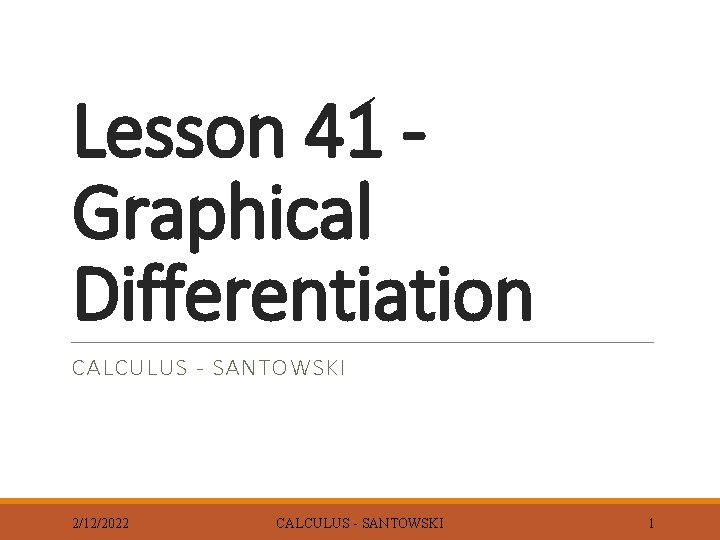Lesson 41 Graphical Differentiation CALCULUS - SANTOWSKI 2/12/2022 CALCULUS - SANTOWSKI 1Lesson Objectives 1. Given the equation of a function, graph it and then make conjectures about the relationship between the derivative function and the original function 2. From a function, sketch its derivative 3. From a derivative, graph an original function 2/12/2022 CALCULUS - SANTOWSKI 2Fast Five 1. Find f(x) if f’(x) = -x 2 + 2 x 2. Sketch a graph whose first derivative is always negative 3. Graph the derivative of the function 4. If the graph represented the derivative, sketch the original function 2/12/2022 CALCULUS - SANTOWSKI 3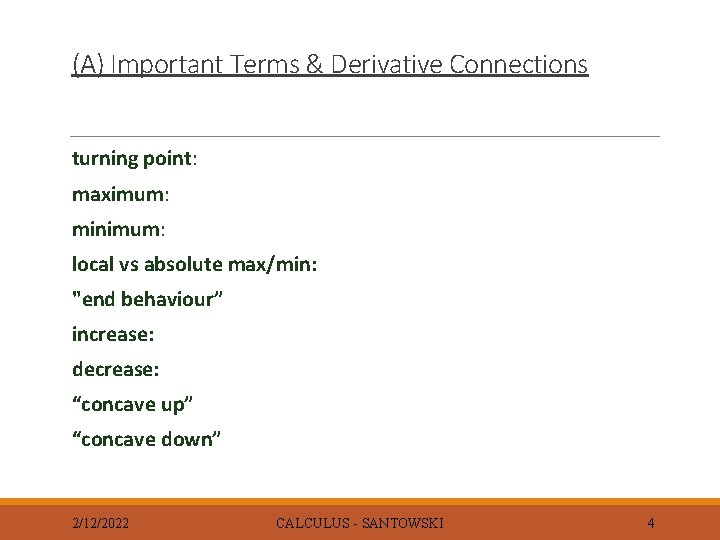(A) Important Terms & Derivative Connections turning point: maximum: minimum: local vs absolute max/min: "end behaviour” increase: decrease: “concave up” “concave down” 2/12/2022 CALCULUS - SANTOWSKI 4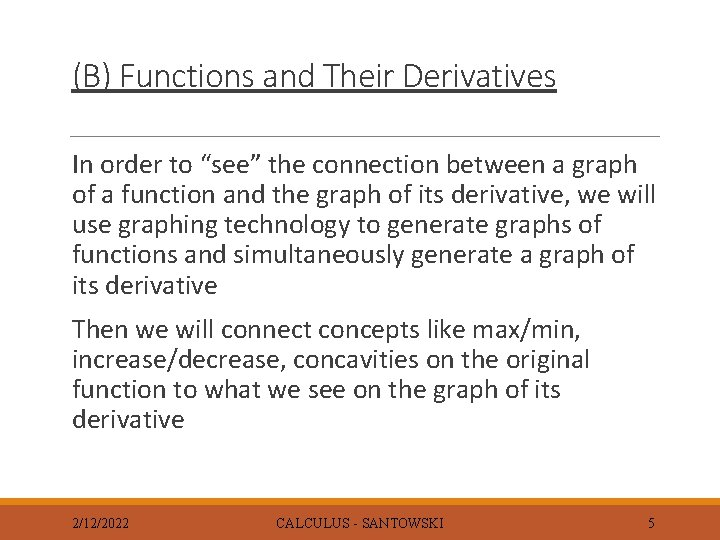(B) Functions and Their Derivatives In order to “see” the connection between a graph of a function and the graph of its derivative, we will use graphing technology to generate graphs of functions and simultaneously generate a graph of its derivative Then we will connect concepts like max/min, increase/decrease, concavities on the original function to what we see on the graph of its derivative 2/12/2022 CALCULUS - SANTOWSKI 5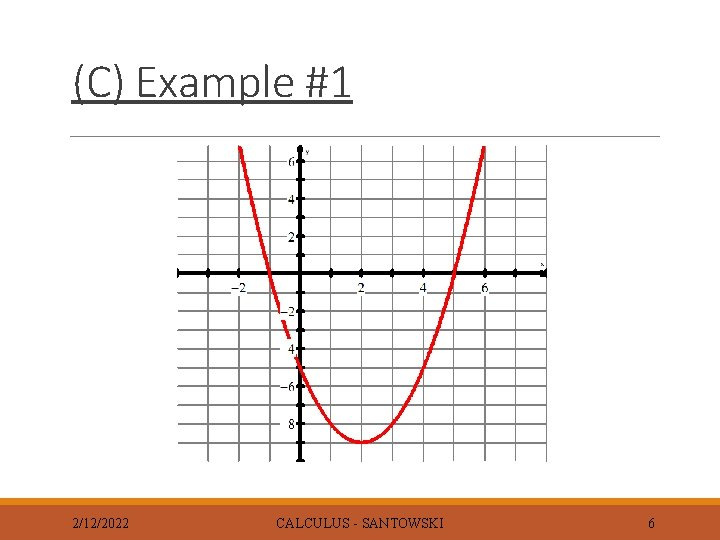(C) Example #1 2/12/2022 CALCULUS - SANTOWSKI 6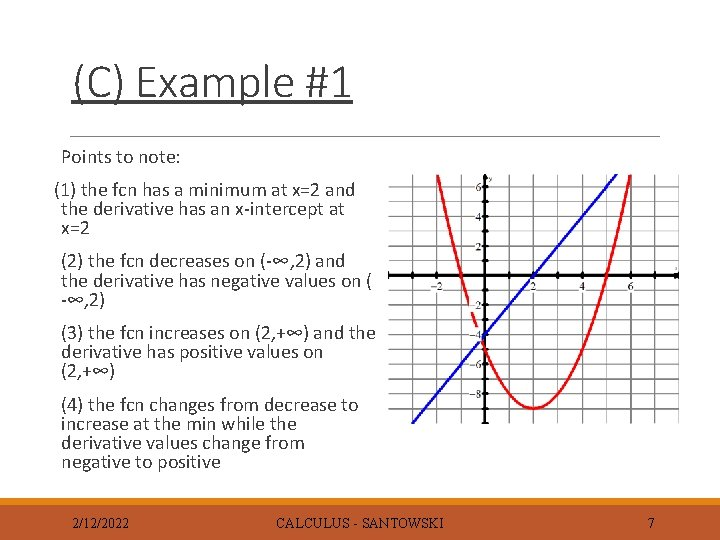(C) Example #1 Points to note: (1) the fcn has a minimum at x=2 and the derivative has an x-intercept at x=2 (2) the fcn decreases on (-∞, 2) and the derivative has negative values on ( -∞, 2) (3) the fcn increases on (2, +∞) and the derivative has positive values on (2, +∞) (4) the fcn changes from decrease to increase at the min while the derivative values change from negative to positive 2/12/2022 CALCULUS - SANTOWSKI 7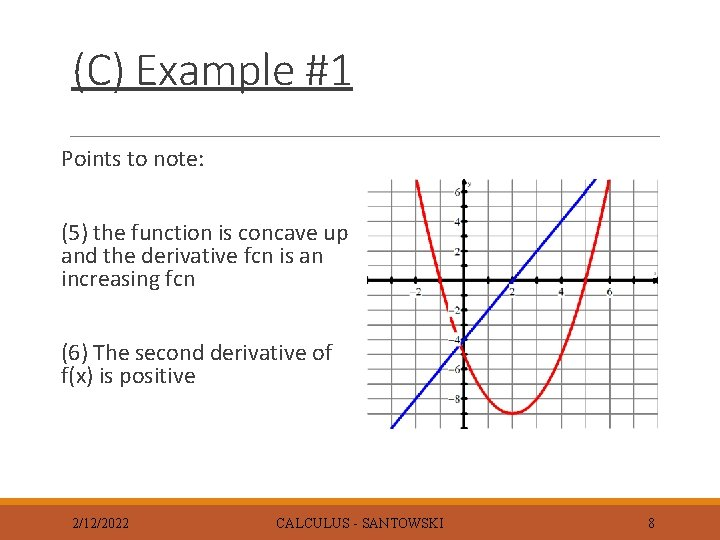(C) Example #1 Points to note: (5) the function is concave up and the derivative fcn is an increasing fcn (6) The second derivative of f(x) is positive 2/12/2022 CALCULUS - SANTOWSKI 8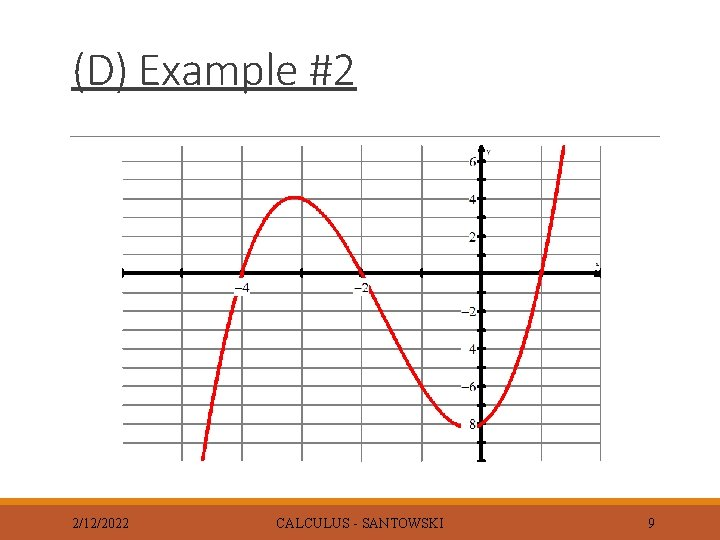(D) Example #2 2/12/2022 CALCULUS - SANTOWSKI 9(D) Example #2 f(x) has a max. at x = -3. 1 and f `(x) has an xintercept at x = -3. 1 f(x) has a min. at x = -0. 2 and f `(x) has a root at – 0. 2 f(x) increases on (- , -3. 1) & (-0. 2, ) and on the same intervals, f `(x) has positive values f(x) decreases on (-3. 1, -0. 2) and on the same interval, f `(x) has negative values At the max (x = -3. 1), the fcn changes from being an increasing fcn to a decreasing fcn the derivative changes from positive values to negative values At a the min (x = -0. 2), the fcn changes from decreasing to increasing the derivative changes from negative to positive 2/12/2022 CALCULUS - SANTOWSKI 10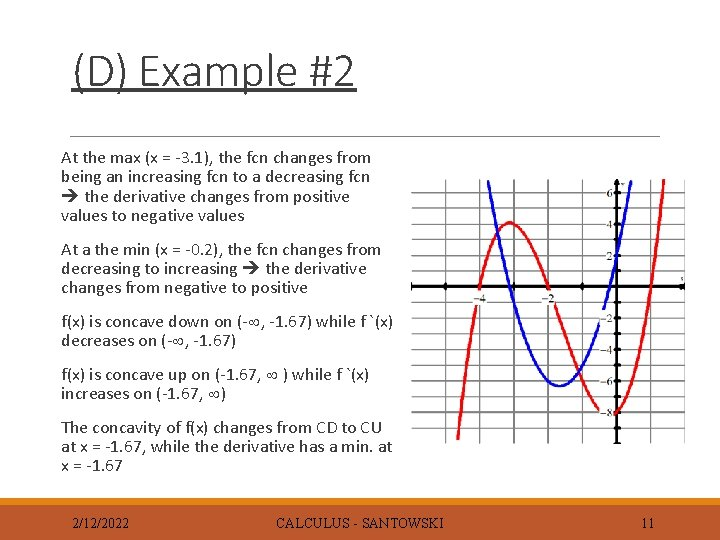(D) Example #2 At the max (x = -3. 1), the fcn changes from being an increasing fcn to a decreasing fcn the derivative changes from positive values to negative values At a the min (x = -0. 2), the fcn changes from decreasing to increasing the derivative changes from negative to positive f(x) is concave down on (- , -1. 67) while f `(x) decreases on (- , -1. 67) f(x) is concave up on (-1. 67, ) while f `(x) increases on (-1. 67, ) The concavity of f(x) changes from CD to CU at x = -1. 67, while the derivative has a min. at x = -1. 67 2/12/2022 CALCULUS - SANTOWSKI 11(E) Sketching Graph of Derivatives from the Graph of a Function Now, we will build upon this thorough analysis of a function & its connection to the derivative in order to (i) predict what derivatives of more complicated functions look like and (ii) work in REVERSE (given a derivative, sketch the original fcn To further visualize the relationship between the graph of a function and the graph of its derivative function, we can run through some exercises wherein we are given the graph of a function can we draw a graph of the derivative and vice versa 2/12/2022 CALCULUS - SANTOWSKI 12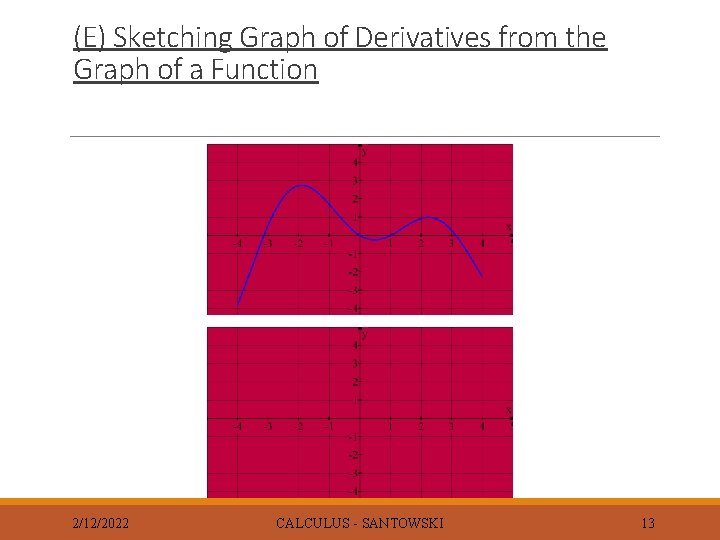(E) Sketching Graph of Derivatives from the Graph of a Function 2/12/2022 CALCULUS - SANTOWSKI 13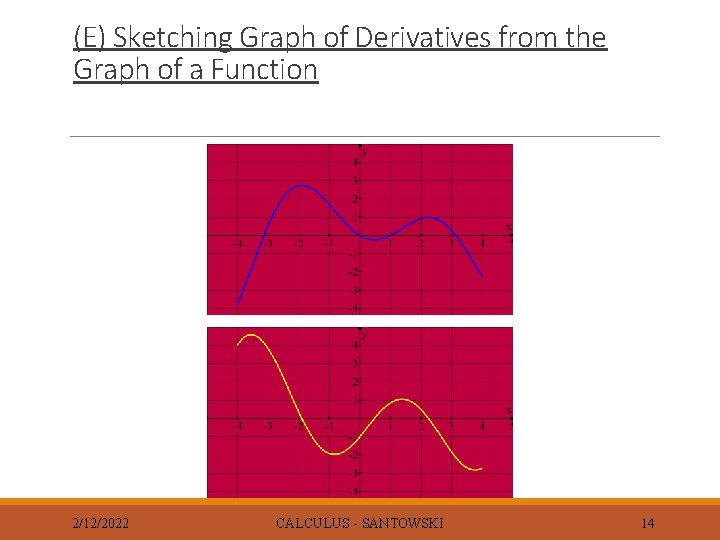(E) Sketching Graph of Derivatives from the Graph of a Function 2/12/2022 CALCULUS - SANTOWSKI 14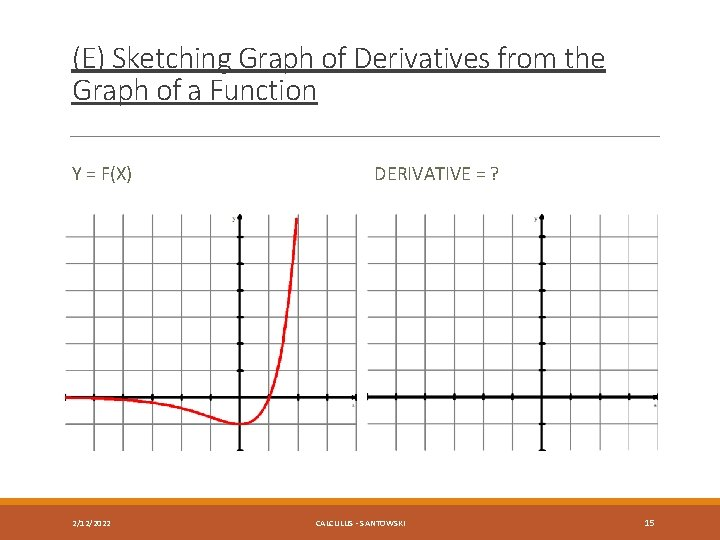(E) Sketching Graph of Derivatives from the Graph of a Function Y = F(X) 2/12/2022 DERIVATIVE = ? CALCULUS - SANTOWSKI 15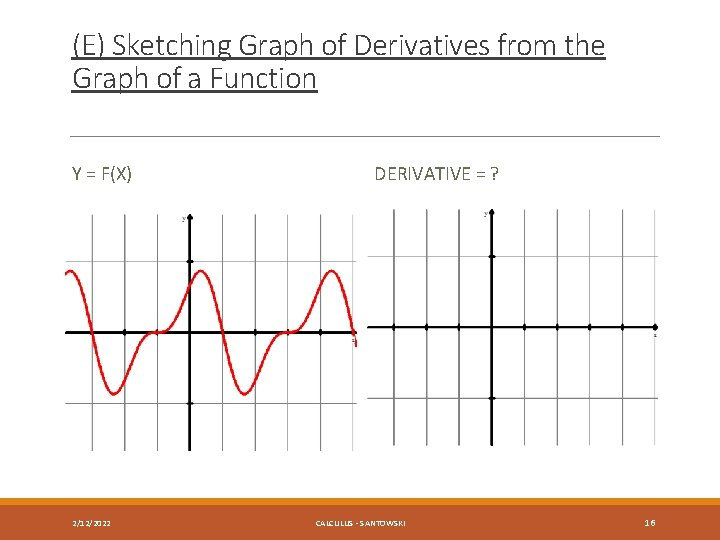(E) Sketching Graph of Derivatives from the Graph of a Function Y = F(X) 2/12/2022 DERIVATIVE = ? CALCULUS - SANTOWSKI 16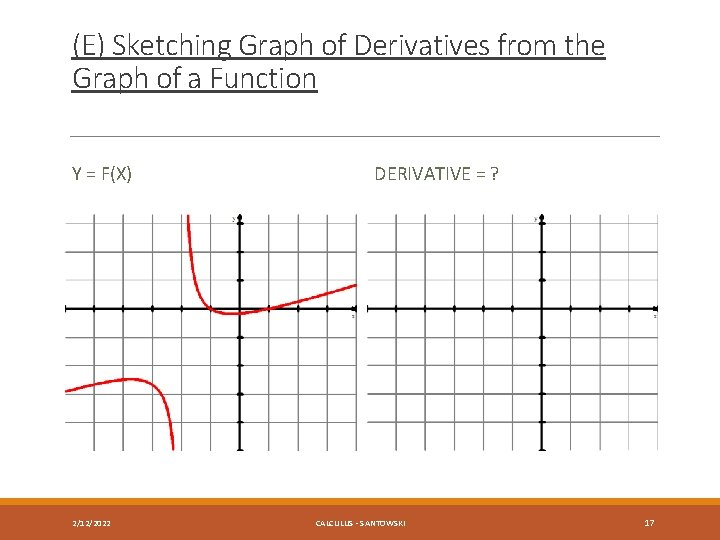(E) Sketching Graph of Derivatives from the Graph of a Function Y = F(X) 2/12/2022 DERIVATIVE = ? CALCULUS - SANTOWSKI 17(F) Sketching a Function from the Graph of its Derivative 2/12/2022 CALCULUS - SANTOWSKI 18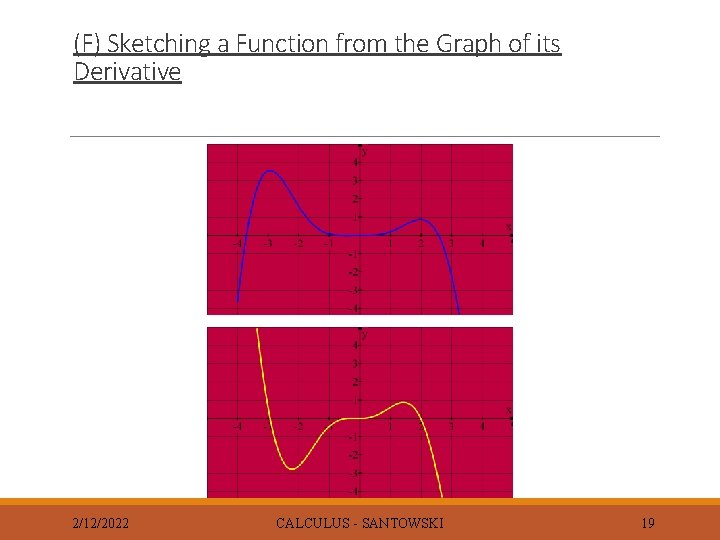(F) Sketching a Function from the Graph of its Derivative 2/12/2022 CALCULUS - SANTOWSKI 19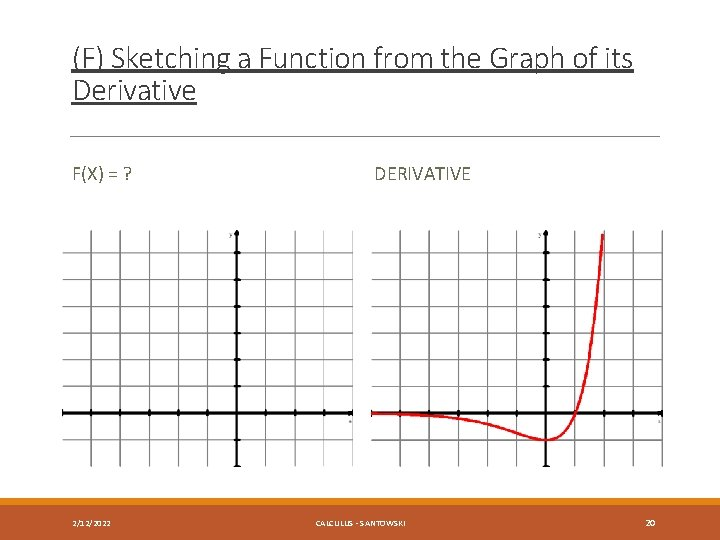(F) Sketching a Function from the Graph of its Derivative F(X) = ? 2/12/2022 DERIVATIVE CALCULUS - SANTOWSKI 20(F) Sketching a Function from the Graph of its Derivative F(X) = ? 2/12/2022 DERIVATIVE CALCULUS - SANTOWSKI 21(F) Sketching a Function from the Graph of its Derivative F(X) = ? 2/12/2022 DERIVATIVE CALCULUS - SANTOWSKI 22(G) Matching Function Graphs and Their Derivative Graphs - Internet Links Work through these interactive applets from maths online Gallery - Differentiation 1 wherein we are given graphs of functions and also graphs of derivatives and we are asked to match a function graph with its derivative graph (http: //www. univie. ac. at/future. media/moe/galerie/di ff 1/diff 1. html) http: //www. univie. ac. at/moe/tests/diff 1/ablerkennen. html 2/12/2022 CALCULUS - SANTOWSKI 23Links https: //www. khanacademy. org/math/differential-calculus/takingderivatives/visualizing-derivatives-tutorial/e/derivative_intuition http: //webspace. ship. edu/msrenault/Geo. Gebra. Calculus/derivative_try _to_graph. html http: //webspace. ship. edu/msrenault/Geo. Gebra. Calculus/derivative_ma tching. html http: //webspace. ship. edu/msrenault/Geo. Gebra. Calculus/derivative_ap p_1_graph_AD. html http: //webspace. ship. edu/msrenault/Geo. Gebra. Calculus/derivative_ma tching_antiderivative. html 2/12/2022 CALCULUS - SANTOWSKI 24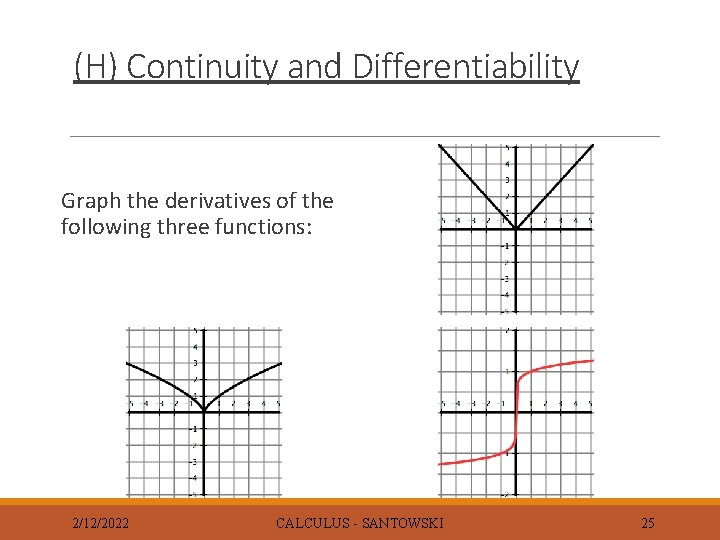(H) Continuity and Differentiability Graph the derivatives of the following three functions: 2/12/2022 CALCULUS - SANTOWSKI 25(H) Continuity and Differentiability Continuous functions are non-differentiable under the following conditions: ◦ The fcn has a “corner” (ex 1) ◦ The fcn has a “cusp” (ex 2) ◦ The fcn has a vertical tangent (ex 3) This non-differentiability can be seen in that the graph of the derivative has a discontinuity in it! 2/12/2022 CALCULUS - SANTOWSKI 26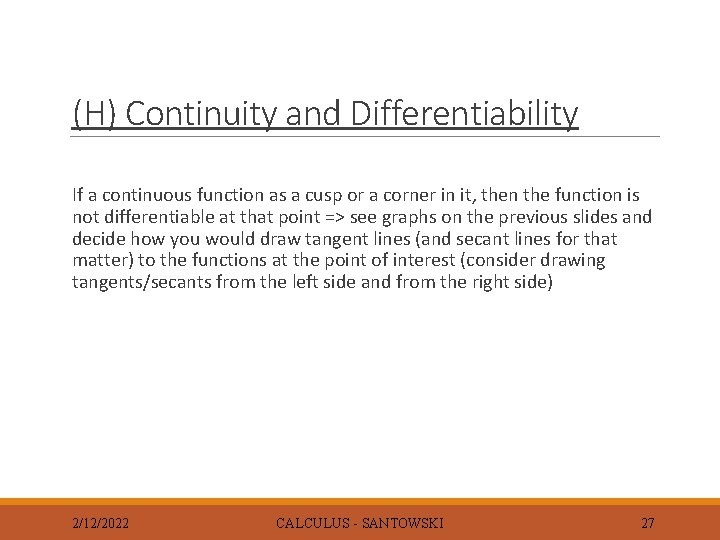(H) Continuity and Differentiability If a continuous function as a cusp or a corner in it, then the function is not differentiable at that point => see graphs on the previous slides and decide how you would draw tangent lines (and secant lines for that matter) to the functions at the point of interest (consider drawing tangents/secants from the left side and from the right side) 2/12/2022 CALCULUS - SANTOWSKI 27